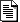Welcome to Gopherspace! You are browsing Gopher through a Web interface right now. You can use most browsers to browse Gopher natively. If your browser supports it, click here to see this page in Gopher directly. To find Gopher browsers, click here.

Gopher: gopher.2001-12[gopher] =?GB2312?Q?net114(=CD=F8=C2=E7114)?= plain[gopher] =?GB2312?Q?net114(=CD=F8=C2=E7114)?= plain[gopher] =?GB2312?Q?net114(=CD=F8=C2=E7114)?= plain[gopher] StarChefs December Newsletter plain[gopher] Re: overriding portions of the http protocol? plain[gopher] Re: overriding portions of the http protocol? plain[gopher] [Paul Lindner ] Re: overriding portions of the http protocol? plain[gopher] Re: overriding portions of the http protocol? plain[gopher] Re: [Paul Lindner ] Re: overriding portions of the http protocol? plain[gopher] Re: [Paul Lindner ] Re: overriding portions of the http protocol? plain[gopher] Re: [Paul Lindner ] Re: overriding portions of the http protocol? plain[gopher] gophervr? plain[gopher] Re: gophervr? plain[gopher] Re: gophervr? plain[gopher] General Gopher question, from a Corporal plain[gopher] Re: [Paul Lindner ] Re: overriding portions of the http protocol? plain[gopher] =?GB2312?Q?=CF=D6=B4=FA=C6=F3=D2=B5=B5=E7=D7=D3=C9=CC=CE=F1=CA=D6=B2=E1?= plain[gopher] =?GB2312?Q?=CD=F8=C9=CF=BB=C6=D2=B3=A3=AC=C3=E2=B7=D1=B5=C7=C2=BC=A3=A1?= plain[gopher] =?GB2312?Q?=CB=EA=C4=A9=B3=AC=D6=B5=B9=BAPALM,=BE=AB=C3=C0=C0=F1=C6=B7=B4=F3=D4=F9=CB=CD=A3=A1?= plain[gopher] Mac archives @ Floodgap and GopherVR plain

[server top] [view with gopher]
Generated by PyGopherd